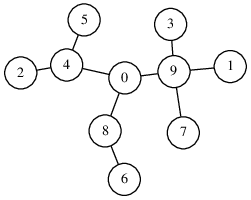Your browser (Unknown 0) is no longer supported. Some parts of the website may not work correctly. Please update your browser.

#### MinRouterPeripherality

For each node in a tree find the sum of distances to all other nodes.

A computer network consisting of N routers and N−1 links connecting them is given. Routers are labeled with distinct integers within the range [0..(N−1)]. Links connect routers in such a way that each distinct pair of routers is connected either by a direct link or through a path consisting of direct links. There is exactly one way to reach any router from another and the number of direct links that must be traversed is called the distance between these two routers. For example, consider the following network consisting of ten routers and nine links:Routers 2 and 4 are connected directly, so the distance between them is 1. Routers 4 and 7 are connected through a path consisting of direct links 4−0, 0−9 and 9−7; hence the distance between them is 3.

The location of a router in the network determines how quickly a packet dispatched by that router can reach other routers. The peripherality of a router is the average distance to all other routers on the network. For example, the peripherality of router 4 in the network shown above is 2.11, because:

 distance to 0: 1 distance to 1: 3 distance to 2: 1 distance to 3: 3 distance to 5: 1 distance to 6: 3 distance to 7: 3 distance to 8: 2 distance to 9: 2 average: 19/9 = 2.11

The peripherality of router 0 is 1.66 and no other router has lower peripherality.

Write a function

class Solution { public int solution(int[] T); }

that, given a non-empty array T consisting of N integers describing a network of N routers and N−1 links, returns the label of the router that has minimum peripherality. If there is more than one router that has minimum peripherality, the function should return the lowest label.

Array T describes a network of routers as follows:

• if T[P] = Q and P ≠ Q, then there is a direct link between routers P and Q.

For example, given the following array T consisting of ten elements:

T = 9 T = 1 T = 4 T = 9 T = 0 T = 4 T = 8 T = 9 T = 0 T = 1

the function should return 0, because this array describes the network shown above and router 0 has minimum peripherality.

Write an efficient algorithm for the following assumptions:

• N is an integer within the range [1..100,000];
• each element of array T is an integer within the range [0..(N−1);
• there is exactly one (possibly indirect) connection between any two distinct routers.